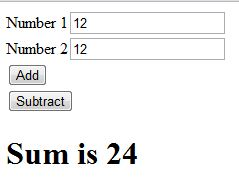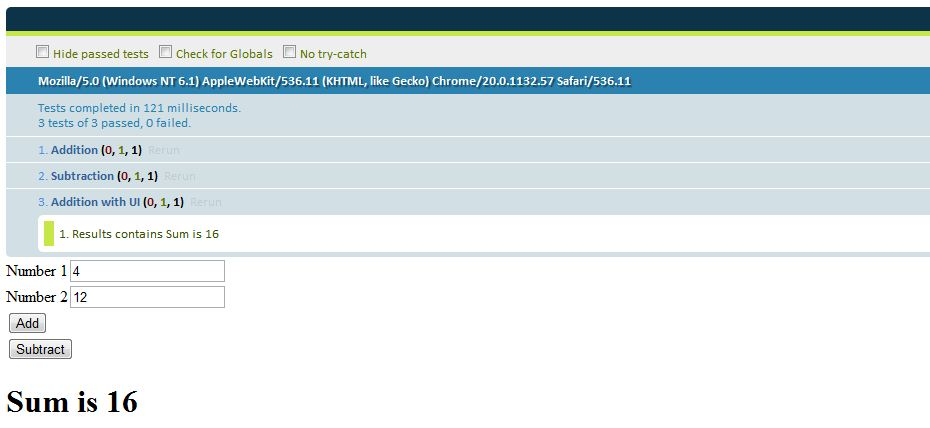# Unit testing JavaScript – III

In the earlier post, we discussed using QUnit to unit-test JavaScript. We didn’t have any UI in the example. Let’s build a simple UI to call the Calculator’s add and subtract functions. Let’s use jQuery to construct the UI as shown below.The calculatortests.html file is shown below.

```<!--calculatortests.html-->
<!DOCTYPE html>
<html>
<head>
<script src="jquery-1.7.2.js"></script>
<script src="calculator.js"></script>
</head>
<body>
Number 1<input type="text" id="num1"><br/>
Number 2<input type="text" id="num2"><br/>
<input type="button"  value="Add" id="addBtn"><br/>
<input type="button"  value="Subtract" id="subtractBtn">
<h1 id="results"></h1>
</body>
</html>
```

Hitting the Add/Subtract button will display the results in h1 element. The Calculator.js file with the UI functionality is shown below:

```\$().ready(function(){
\$("#addBtn").on("click",function(){
var results = add(parseInt(\$("#num1").val()),parseInt(\$("#num2").val()));
\$("#results").html("Sum is " + results);
});
\$("#subtractBtn").on("click",function(){
var results = subtract(parseInt(\$("#num1").val()),parseInt(\$("#num2").val()));
\$("#results").html("Difference is " + results);
});
});
function add(a,b){
return a+b;
}
function subtract(a,b){
return a-b;
}
```

We’ll now create a calculatortests.js that has the test for add and subtract functions. This file will also have the test to display the results in h1 element when the buttons are clicked.

```test("Addition",function(){
equal(4,add(2,2),"2+2 is 4");
});
test("Subtraction",function(){
equal(4,subtract(6,2),"Difference of 6,2 is 4");
});
test("Addition with UI",function(){
\$("#num1").val(4);
\$("#num2").val(12);
\$("#addBtn").trigger("click");
equal(\$("#results").text(),"Sum is 16","Results contains Sum is 16");
});
```

What’s interesting is the test “Addition with UI”. We set the values in the textboxes explicility and programmatically trigger the click event of the button. We test if the h1 element results contains the expected output.

Let’s include calculatortests.js,qunit.js, qunit.css and also a div element with id as “qunit in the calculatortests.html. Executing the page gives you the following output.As you can see, testing jQuery code using qunit is pretty easy as well. The “trigger” function does the magic. How about having some AJAX calls in our code?Let’s look at it in the next post.